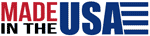## Discount Electronic Components

Need assistance? Give us a call. (631) 234-3857

# Allen Bradley Carbon Composition Resistors

There have been rumors that were spread throughout the electronic components market place that there was no more 2 Watt Allen Bradley Carbon Comp Resistors. However Allen Bradley famous American-made real carbon composition resistors still DO exist.

TEDSS.com has in stock, in its 100,000 square foot inventory, over 2,107,533 of these resistors, ready to ship. This constitutes the world’s largest supply of Allen Bradley 1/8, 1/4, 1/2, 1, and 2 Watt resistors, designated by the MIL-R-11B, MIL-R-39008, MS35045-00, RC05 / RCR05, RC07 / RCR07, RC20 / RCR20, RC32 / RCR32 and RC42 / RCR42 (for established reliability) respectively.

You can stop searching for your resistors and visit Tedss.com

## Allen Bradley Carbon Composition Resistors Specification.

The standard resistance range of these superior hot molded carbon composition resistors is 1ohm up to 22Mohm (22,000,000 ohm).

Temperature range works up to 70° C. The leads are oxygen-free copper leads.

These rugged, low-shocks, low-vibration resistors can be ordered in 5% tolerance (color code gold) or 10% tolerance (color code silver).

## MIL-R-39008 Type Part Number Designation:

RCR 20 G 514 J S

RCR 20 G 514 J S
| | | | | |
Type Style / Wattage Temperature Resistance Code Tolerance Failure Level

Type:

• RCR MIL-R-39008 Type Designation, Fixed Composition Resistors, Insulated, Established Reliability.

Style / Wattage:

• 05 - 1/8 Watt
• 07 - 1/4 Watt
• 20 - 1/2 Watt
• 32 - 1 Watt
• 42 - 2 Watt

Maximum Ambient Temperature and Resistance Temperature Characteristic:

• Refer to MIL-R-39008 specification

Resistance Code:

• Expressed in ohms and identified by a three-digit number.
• First two digits represent significant figures.
• Last digit specifies the number of zeros to follow (Example 223 = 22,000 ohms = 22 Kohms), except below 10 ohms.
• The letter "R" is substituted in place of a significant digit to represent the decimal point. (Example 2R7 = 2.7 ohms)

Tolerance:

• J = 5%
• K = 10%

Failure Level:

• At 50% rated wattage expressed in %/1000 hrs.
• M = 1.0%
• P = 0.1%
• R = 0.01%
• S = 0.001%

## MIL-R-11 Type Part Number Designation:

RC 20 GF 514 J

RC 20 GF 514 J
| | | | |
Type Style / Wattage Temperature Resistance Code Tolerance

Type:

• RC MIL-R-11 Type Designation

Style / Wattage:

• 05 - 1/8 Watt
• 07 - 1/4 Watt
• 20 - 1/2 Watt
• 32 - 1 Watt
• 42 - 2 Watt

Maximum Ambient Temperature and Resistance Temperature Characteristic:

• Refer to MIL-R-11 specification

Resistance Code:

• Expressed in ohms and identified by a three-digit number.
• First two digits represent significant figures.
• Last digit specifies the number of zeros to follow (Example 223 = 22,000 ohms = 22 Kohms), except below 10 ohms.
• The letter "R" is substituted in place of a significant digit to represent the decimal point. (Example 2R7 = 2.7 ohms)

Tolerance:

• J = 5%
• K = 10%

## Industrial Type Part Number Designation:

EB 514 5

EB 514 5
| | |
Style / Wattage Resistance Code Tolerance

Style / Wattage:

• BB - 1/8 Watt
• CB - 1/4 Watt
• EB - 1/2 Watt
• GB - 1 Watt
• HB - 2 Watt

Resistance Code:

• Expressed in ohms and identified by a three-digit number.
• First two digits represent significant figures.
• Last digit specifies the number of zeros to follow (Example 223 = 22,000 ohms = 22 Kohms), except below 10 ohms.
• The letter "G" is substituted in place of a third digit. The desired resistance value is then the first two digits multiplied by 0.1 (Example 27G = 2.7 ohms)

Tolerance:

• 5 = 5%
• 1 = 10%
• 2 = 10%

## Allen Bradley Carbon Composition Resistors.

These American-made carbon composition resistors were made in Wisconsin. These resistors are extremely rugged due to the hot molding process. The A-B resistors are highly recognized for their outstanding MIL-SPEC reliability, pulse withstanding, power dissipation, high frequency stability, low inductance, rugged construction and dependable performance.